Home | | Computer Science 11th std | Lexical Units (Tokens) - C++ program

# Lexical Units (Tokens) - C++ program

Lexical elements or Tokens. C++ has the following tokens: • Keywords • Identifiers • Literals • Operators • Punctuators

Lexical Units (Tokens):

C++ program statements are constructed by many different small elements such as commands, variables, constants and many more symbols called as operators and punctuators. These individual elements are collectively called as Lexical units or Lexical elements or Tokens. C++ has the following tokens:

• Keywords

• Identifiers

• Literals

• Operators

• Punctuators

TOKEN:

The smallest individual unit in a program is known as a Token or a Lexical unit

## 1. Keywords

Keywords are the reserved words which convey specific meaning to the C++ compiler. They are the essential elements to construct C++ programs. Most of the keywords are common to C, C++ and Java.

C++ is a case sensitive programming language so, all the keywords must be in lowercase.

Table 9.1 C++ Keywords·           With revisions and additions, the recent list of keywords also includes:

using, namespace, bal, static_cast, const_cast, dynamic_cast, true, false

·           Identifiers containing a double underscore are reserved for use by C++ implementations and standard libraries and should be avoided by users.

## 2. Identifiers

Identifiers are the user-defined names given to different parts of the C++ program viz. variables, functions, arrays, classes etc., These are the fundamental building blocks of a program. Every language has specific rules for naming the identifiers.

### Rules for naming an identifier:

• The first character of an identifier must be an alphabet or an underscore (_).Only alphabets, digits and underscore are permitted. Other special characters are not allowed as part of an identifier.C++ is case sensitive as it treats upper and lower-case characters differently.Reserved words or keywords cannot be used as an identifier name.

As per ANSI standards, C++ places no limit on its length and therefore all the characters are significant.• You may use an underscore in variable names to separate different parts of the name (eg: total_sales is a valid identifier where as the variable called total sales is an invalid identifier).

• You may use capital style notation, such as tamilMark ie. capitalizing the first letter of the second word.

## 3. Literals (Constants)

Literals are data items whose values do not change during the execution of a program. Therefore Literals are called as Constants. C++ has several kinds of literals:### Numeric Constants:

As the name indicates, the numeric constants are numeric values, which are used as constants. Numeric constants are further classified as:

• Integer Constants (or) Fixed point constants.

• Real constants (or) Floating point constants.

### (1) Integer Constants (or) Fixed point constants

Integers are whole numbers without any fractions. An integer constant must have at least one digit without a decimal point. It may be signed or unsigned. Signed integers are considered as negative, commas and blank spaces are not allowed as part of it. In C++, there are three types of integer constants: (i) Decimal (ii) Octal (iii) Hexadecimal

### (i) Decimal

Any sequence of one or more digits (0 …. 9)If you assign 4.56 as an integer decimal constant, the compiler will accept only the integer portion of 4.56 ie. 4. It will simply ignore .56.

If a Decimal constant declared with fractions, then the compiler will take only the integer part of the value and it will ignore its fractional part. This is called as “Implicit Conversion”. It will be discussed later.

### (ii) Octal

Any sequence of one or more octal values (0 …. 7) that begins with 0 is considered as an Octal constant.When you use a fractional number that begins with 0, C++ has consider the number as an integer not an Octal.

Any sequence of one or more Hexadecimal values (0 …. 9, A …. F) that starts with 0x or 0Xis considered as an Hexadecimal constant.The suffix L or l and U or u added with any constant forces that to be represented as a long or unsigned constant respectively.

### (2) Real Constants (or) Floating point constants

A real or floating point constant is a numeric constant having a fractional component. These constants may be written in fractional form or in exponent form.

Fractional form of a real constant is a signed or unsigned sequence of digits including a decimal point between the digits. It must have at least one digit before and after a decimal point. It may have prefix with + or - sign. A real constant without any sign will be considered as positive.

Exponent form of real constants consists of two parts: (1) Mantissa and (2) Exponent. The mantissa must be either an integer or a real constant. The mantissa followed by a letter E or e and the exponent. The exponent should also be an integer.

For example, 58000000.00 may be written as 0.58 × 108 or 0.58E8.### Example:### Boolean Literals

Boolean literals are used to represent one of the Boolean values(True or false). Internally true has value 1 and false has value 0.

### Character constant

A character constant is any valid single character enclosed within single quotes. A character constant in C++ must contain one character and must be enclosed within a single quote.

Valid character constants : ‘A’, ‘2’, ‘\$’

Invalid character constants : “A”

The value of a single character constant has an equivalent ASCII value. For example, the value of ‘A’ is 65.

### Escape sequences (or) Non-graphic characters

C++ allows certain non-printable characters represented as character constants. Non-printable characters are also called as non-graphical characters. Non-printable characters are those characters that cannot be typed directly from a keyboard during the execution of a program in C++, for example: backspace, tabs etc. These non-printable characters can be represented by using escape sequences. An escape sequence is represented by a backslash followed by one or two characters.

Table 9.2 Escape Sequences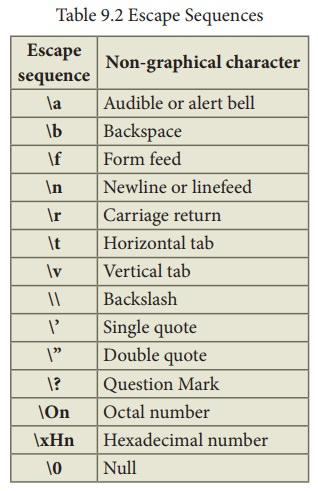Even though an escape sequence contains two characters, they should be enclosed within single quotes because, C++ consider escape sequences as character constants and allocates one byte in ASCII representation.

ASCII (American Standard Code for Information Interchange) was first developed and published in 1963 by the X3 committee, a part of the American Standards Association (ASA).

### String Literals

Sequence of characters enclosed within double quotes are called as String literals. By default, string literals are automatically added with a special character ‘\0’ (Null) at the end. Therefore, the string “welcome” will actually be represented as “welcome\0” in memory and the size of this string is not 7 but 8 characters i.e., inclusive of the last character \0.

Valid string Literals : “A”, “Welcome” “1234”

Invalid String Literals : ‘Welcome’, ‘1234’

## 4. Operators

The symbols which are used to do some mathematical or logical operations are called as “Operators”. The data items or values that the operators act upon are called as “Operands”.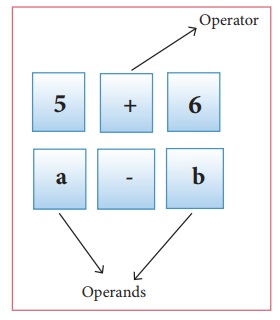In C++, The operators are classified on the basis of the number of operands.

(i) Unary Operators - Require only one operand

(ii) Binary Operators - Require two operands

(iii) Ternary Operators - Require three operands

## C++ Operators are classified as:

(1) Arithmetic Operators

(2) Relational Operators

(3) Logical Operators

(4) Bitwise Operators

(5) Assignment Operators

(6) Conditional Operator

(7) Other Operators

### (1) Arithmetic Operators

Arithmetic operators perform simple arithmetic operations like addition, subtraction, multiplication, division etc.,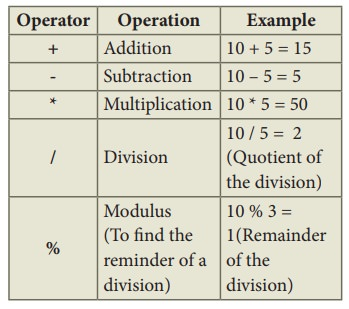• The above mentioned arithmetic operators are binary operators which requires minimum of two operands.

### Increment and Decrement Operators

++ (Plus, Plus) Increment operator

-- (Minus, Minus) Decrement operator

An increment or decrement operator acts upon a single operand and returns a new value. Thus, these operators are unary operators. The increment operator adds 1 to its operand and the decrement operator subtracts 1 from its operand. For example,

• x++ is the same as x = x+1;

It adds 1 to the present value of x

• x-- is the same as to x = x–1;

It subtracts 1 from the present value of x

The ++ or -- operators can be placed either as prefix (before) or as postfix (after) to a variable. With the prefix version, C++ performs the increment / decrement before using the operand.For example:  N1=10, N2=20;

S = ++N1 + ++N2;

The following Figure explains the working process of the above statement.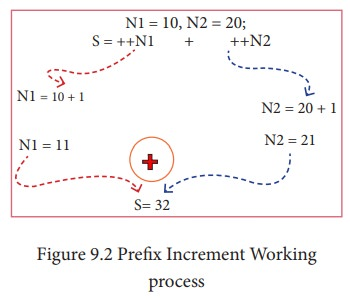In the above example, the value of num is first incremented by 1, then the incremented value is assigned to the respective operand.

With the postfix version, C++ uses the value of the operand in evaluating the expression before incrementing / decrementing its present value.

For example: N1=10, N2=20;

S = N1++ + ++N2;

The following Figure explains the working process of the above statement.In the above example, the value assigned to operand N1 is taken into consideration, first and then the value will be incremented by 1.### (2) Relational Operators

Relational operators are used to determine the relationship between its operands. When the relational operators are applied on two operands, the result will be a Boolean value i.e 1 or 0 to represents True or False respectively. C++ provides six relational operators. They are,• In the above examples, the operand a is compared with b and depending on the relation, the result will be either 1 or 0. i.e., 1 for true, 0 for false.

• All six relational operators are binary operators.

### (3) Logical Operators

A logical operator is used to evaluate logical and relational expressions. The logical operators act upon the operands that are themselves called as logical expressions. C++ provides three logical operators.• AND, OR both are binary operators where as NOT is an unary operator.

Example: a = 5, b = 6, c = 7;### (4)Bitwise Operators

Bitwise operators work on each bit of data and perform bit-by-bit operation. In C++, there are three kinds of bitwise operators, which are:

(i) Logical bitwise operators

(ii) Bitwise shift operators

(iii) One’s compliment operators

### (i) Logical bitwise operators:

& Bitwise AND (Binary AND)

| Bitwise OR (Binary OR)

^ Bitwise Exclusive OR (Binary XOR)

• Bitwise AND (&) will return 1 (True)if both the operands are having the value 1 (True); Otherwise, it will return 0 (False)

• Bitwise OR (|) will return 1 (True) if any one of the operands is having a value 1 (True); It returns 0 (False) if both the operands are having the value 0 (False)

• Bitwise XOR (^) will return 1 (True) if only one of the operand is having a value 1 (True). If both are True or both are False, it will return 0 (False).

Truth table for bitwise operators (AND, OR, XOR)Example:

If a = 65, b=15

Equivalent binary values of 65 = 0100 0001; 15 = 0000 1111### (ii) The Bitwise shift operators:

There are two bitwise shift operators in C++, Shift left (<<) and Shift right (>>).

Shift left ( << )– The value of the left operand is moved to left by the number of bits specified by the right operand. Right operand should be an unsigned integer.

Shift right ( >> )– The value of the left operand is moved to right by the number of bits specified by the right operand. Right operand should be an unsigned integer.

### Example:

If a =15; Equivalent binary value of a is 0000 1111### (iii) The Bitwise one’s compliment operator:

The bitwise One’s compliment operator ~(Tilde),inverts all the bits in a binary pattern, that is, all 1’s become 0 and all 0’s become 1. This is an unary operator.

### Example:

If a =15; Equivalent binary values of a is 0000 1111### (5)Assignment Operator:

Assignment operator is used to assign a value to a variable which is on the left hand side of an assignment statement. = (equal to) is commonly used as the assignment operator in all computer programming languages. This operator copies the value at the right side of the operator to the left side variable. It is also a binary operator.C++ uses different types of assignment operators. They are called as Shorthand assignment operators.### (6) Conditional Operator:

In C++, there is only one conditional operator is used. ?: is a conditional Operator. This is a Ternary Operator. This operator is used as analternate to if … else control statement. We will learn more about this operator in later chapters along with if …. else structure.

### (7)Other Operators:### Precedence of Operators:

Operators are executed in the order of precedence. The operands and the operators are grouped in a specific logical way for evaluation. This logical grouping is called as an Association.

### The order of precedence:In C++, one or two operators may be used in different places with different meaning.

For example: Asterisk ( * ) is used for multiplication as well as for pointer to a variable.

## 5. Punctuators

Punctuators are symbols, which are used as delimiters, while constructing a C++ program. They are also called as “Separators”. The following punctuators are used in C++; most of these symbols are very similar to C and Java.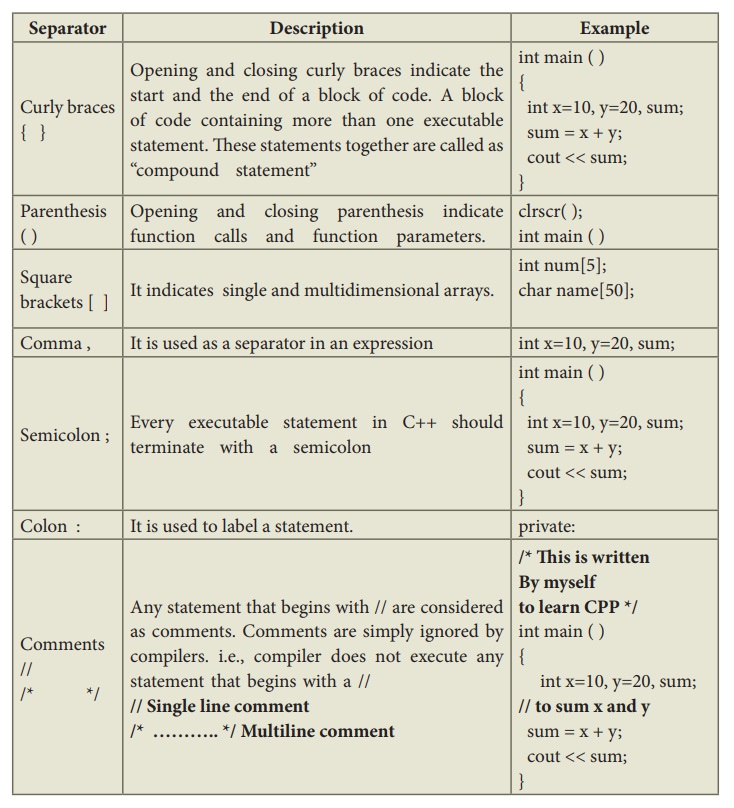Study Material, Lecturing Notes, Assignment, Reference, Wiki description explanation, brief detail
11th Computer Science : Chapter 9 : Introduction to C++ : Lexical Units (Tokens) - C++ program |